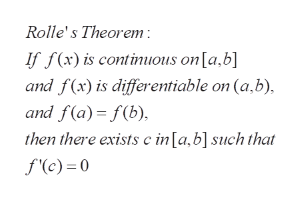# For the given function, find all values of c over the interval (1/2,20) stipulated by Rolle's Theorem such that f′(c)=0. f(x)=x+10x^-1 Submit multiple values as a comma-separated list. Do not round any value.

Question

For the given function, find all values of c over the interval (1/2,20) stipulated by Rolle's Theorem such that f′(c)=0.

f(x)=x+10x^-1

Submit multiple values as a comma-separated list. Do not round any value.

check_circle

Step 1

To solve for all the points c in the given interval satisfying f'(c)=0

Step 2

Statement of Rolle's theorem. Note that all the conditions are satisfied for f(x) over [0.5,20], as the only disco...help_outlineImage TranscriptioncloseRolle's Theorem If fx) is continuous on [a.b] and f(x) is differentiable on (a.b), and f(a) f(b) then there exists c in [a,b] such that f'(c)= 0 fullscreen

### Want to see the full answer?

See Solution

#### Want to see this answer and more?

Solutions are written by subject experts who are available 24/7. Questions are typically answered within 1 hour.*

See Solution
*Response times may vary by subject and question.
Tagged in

### Math